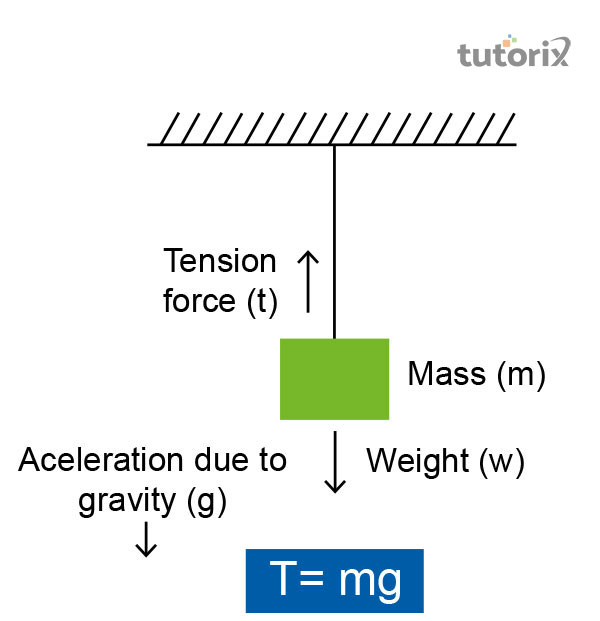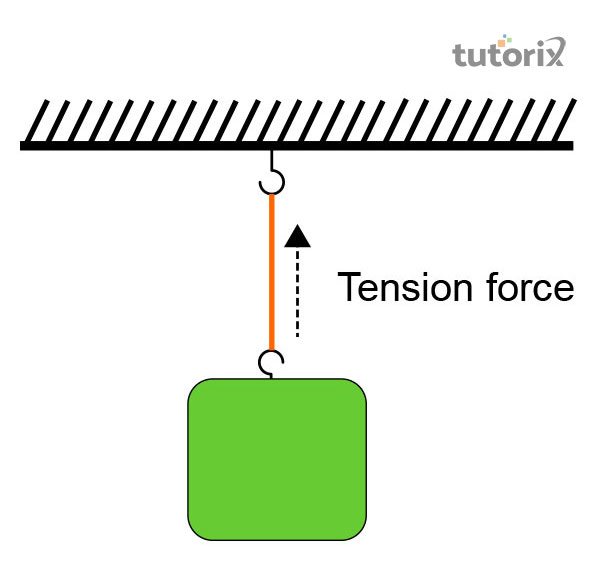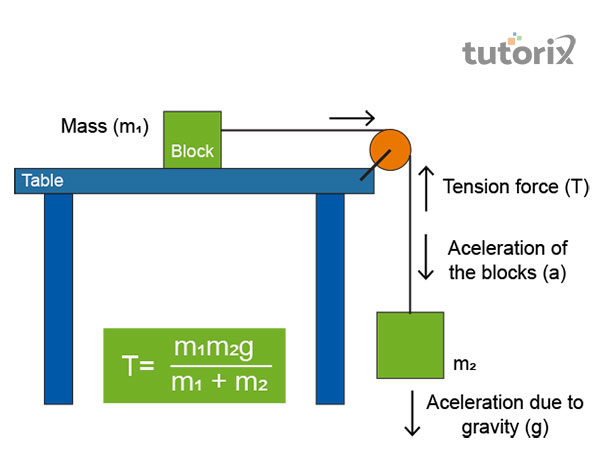# Tension

## Introduction

The force of tension can be either negative or positive and this phenomenon completely depends on the placement area of the coordinated axes. Regardless of the direction that is moving upward which can be taken as negative or positive, the balanced equation for tension force will give an equal result for both positive and negative equations.

In physics tension is a kind of force that is generated due to the application of load at one or more than one end of an object in a directional way. This force that has been generated normally is denoted as the force of tension to the cross-section of the object.

### What do you mean by tension in physics?

In physics tension is referred to the force along the length of an object and this force is carried by a flexible object like cable, wire, or rope. Tension can also be denoted as the action and reaction pair of forces that acts at every end of the flexible elements like wire, cable, or rope (Sciencefacts, 2022).

If a rope is considered as the element then the force of tension will be felt by each section of rope in both directions apart from its endpoints. In the case of the rope, the tension will be experienced at one side of the rope’s endpoints and from the side where the weight is attracted the force will be experienced (Pressbooks, 2022).

The formula of tension can be stated as

Here, T is tension and highlights the condition, T= tension ($\mathrm{N\:or\:kg-m/s^2\:}$) g = acceleration due to gravity $\mathrm{\lgroup9.8 m/s^2\rgroup}$.

### The common misconception about tension

The tension can be considered as the pulling force but not the pushing force. This can be seen in the situation where a rope is hanging from the ceiling where the rope cannot push effectively and is not considered to have the pushing force (Phys. libretexts, 2022).

If an attempt of pushing the rope is made, then it will cause the rope to go slack and lose the tension that its possess.Figure 1: Concept of Tension

People often wrongfully illustrate the force that is acting on a flexible material like wire, or rope as going in the wrong direction. Thus, it is very much vital to keep in mind that force of tension can only pull on and cannot push against the object.

### What is tension force?

In physics the force of tension is determined to be the pulling force where the load upon a subject are applied axially that is on its one or more than one end. The state of stress in which an object is pulled apart is called tension in physics. For example, it can be stated that a wire is attached to the ceiling and has a fixed weight to its lower-end (Dewwool, 2022). There are several types of stress that an object can experience such as shear stress, tensile stress, and normal stress.Figure 2: The force of Tensiond

The major differences between tensile and shear stress is that shear stress deals with the condition where the deforming forces are applied parallel to the surface of the object. Tensile stress deals with the condition where the deforming forces are applied at right angles to the object's surface (Hu et al. 2022).

The examples of the tension‘are suspension bridge, plucking of guitar strings, an elevator that is suspended by a cable, and so on.

### Calculation of the force of tension

The formula of the tension can be written in the form: . The unit of tension is N (Newton) (Yao et al. 2018).

If a block of an object is determined as $\mathrm{m_1}$ and is found lying on horizontal table then $\mathrm{m_1}$ can be pulled by $\mathrm{m_2}$ with the help of a pulley as shown in the picture below.Figure 3: Tension force formula

The 2 blocks of objects will be moving with acceleration a and as per Newton’s 2nd Law, in the case of $\mathrm{m_2}$, $\mathrm{m_2a\:=\:m_2g\:-\:T\:}$ and for $\mathrm{m_1}$, $\mathrm{m_1 a\:=\:T}$. In order to solve the acceleration, the expression $\mathrm{a =\frac{𝐦_2 g}{m_1+m_2}}$ is used.

## Conclusion

The direction of the force of tension is the pulling force and not the pushing force and thus it will point away from the object in direction of the rope or the string. In the case of any hanging flexible object, if the rope pulls the object upwards it exerts an upper pressure on the object and its tension will also be on the upper side. Here the rope is acting as the transferring agent of force. If the two objects are connected with the same rope then both the object's magnitudes of tension force will be different from each other.

### FAQs

Q1. What is tension?

The tension force or tension is the force that is basically experienced by flexible materials like rope, cable or a string. The tension forceis mainly experienced by the object when it is pulled from the opposite end and acts along the rope’s length and is opposite and equal to the applied forced.

Q2. What are proper examples of the force of tension?

The force of tension of the flexible objects basically balances the applied force. The force is only transmitted through a wire when it is pulled otherwise on pushing the wire the results will be in slack. The major examples of the force of tension are the suspension of a pendulum bob, Spring in a watch, swing in the playground, seat belts, and so on

Q3. What will the tension in a string be if 2 same forces of 5 N is put to their end?

There is 10 N of tension force in a string. If the string is stretched by the same force of 5 N in the opposite direction then it will give rise to a tension of 5+5 = 10 N.

Q4. How the force of tension is gets affected by gravity?

The force of tension acts opposite to the gravitational force. A hanging object must be balanced the tension force or else it will get accelerated down because of the gravitational force